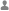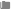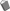## Python导入模块的几种姿势(常规导入, 相对导入, 可选导入, 本地导入)阿里云代理分类：云 知 识 库11已被围观 11次

import sys

import os, sys, time

import sys as system

print(system.platform)

import urllib.error

from functools import lru_cache

functools.lru_cache(*args)

**from os import ***

from os import path, walk, unlink
from os import uname, remove

from os import (path, walk, unlink, uname,
remove, rename)

from os import path, walk, unlink, uname, \
remove, rename

PEP 328介绍了引入相对导入的原因，以及选择了哪种语法。具体来说，是使用句点来决定如何相对导入其他包或模块。这么做的原因是为了避免偶然情况下导入标准库中的模块产生冲突。这里我们以PEP 328中给出的文件夹结构为例，看看相对导入是如何工作的：
my_package/
__init__.py
subpackage1/
__init__.py
module_x.py
module_y.py
subpackage2/
__init__.py
module_z.py
module_a.py

from . import subpackage1
from . import subpackage2

from . import module_x
from . import module_y

from .module_y import spam as ham

def main():
ham()

def spam():
print('spam ' * 3)

In : import my_package

In : my_package.subpackage1.module_x
Out: <module 'my_package.subpackage1.module_x'="" from="" 'my_package="" subpackage1="" module_x.py'="">

In : my_package.subpackage1.module_x.main()
spam spam spam

from . module_y import spam as ham

def main():
ham()

if __name__ == '__main__':
# This won't work!
main()

python module_x.py

Traceback (most recent call last):
File "module_x.py", line 1, in
from . module_y import spam as ham
ValueError: Attempted relative import in non-package

Traceback (most recent call last):
File "module_x.py", line 1, in
from . module_y import spam as ham
SystemError: Parent module '' not loaded, cannot perform relative import

import sys
sys.path.append('/path/to/folder/containing/my_package')
import my_package

try:
# For Python 3
from http.client import responses
except ImportError:  # For Python 2.5-2.7
try:
from httplib import responses  # NOQA
except ImportError:  # For Python 2.4
from BaseHTTPServer import BaseHTTPRequestHandler as _BHRH
responses = dict([(k, v) for k, v in _BHRH.responses.items()])
lxml包也有使用可选导入方式：

try:
from urlparse import urljoin
from urllib2 import urlopen
except ImportError:
# Python 3
from urllib.parse import urljoin
from urllib.request import urlopen

import sys  # global scope

def square_root(a):
# This import is into the square_root functions local scope
import math
return math.sqrt(a)

def my_pow(base_num, power):
return math.pow(base_num, power)

if __name__ == '__main__':
print(square_root(49))
print(my_pow(2, 3))

# a.py
import b

def a_test():
print("in a_test")
b.b_test()

a_test()

import a

def b_test():
print('In test_b"')
a.a_test()

b_test()

import math

def square_root(number):
return math.sqrt(number)

square_root(72)

Traceback (most recent call last):
File "math.py", line 1, in
import math
File "/Users/michael/Desktop/math.py", line 6, in
square_root(72)
File "/Users/michael/Desktop/math.py", line 4, in square_root
return math.sqrt(number)
AttributeError: module 'math' has no attribute 'sqrt'

?